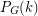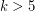Importance: Medium ✭✭
 Author(s): Welsh, Dominic J. A.
 Subject: Graph Theory » Coloring » » Nowhere-zero flows
 Keywords: flow polynomial nowhere-zero flow
 Posted by: mdevos on: March 7th, 2007
Conjecture   All real roots of nonzero flow polynomials are at most 4.

For every graph, letbe the chromatic polynomial ofand letbe the flow polynomial of. Ifis loopless, thenfor all sufficiently large integers(as= # of k-colorings of). It follows from Seymour's 6-flow theorem that ifhas no bridge, thenfor all integers(as= # of nowhere-zero flows in the group of integers modulo). It is natural to ask if all real roots of these polynomials are small. For the chromatic polynomial,, this is not the case. There exist graphs with chromatic number 3 for whichhas arbitrarily large real roots. The above conjecture asserts that the flow polynomial exhibits the opposite behavior. One word of caution, it is known that the set of roots of flow polynomials is dense in the complex plane.

## Bibliography

[S] P.D. Seymour, Nowhere-Zero 6-Flows, J. Combinatorial Theory Ser. B 30 (1981) 130-135. MathSciNet

* indicates original appearance(s) of problem.